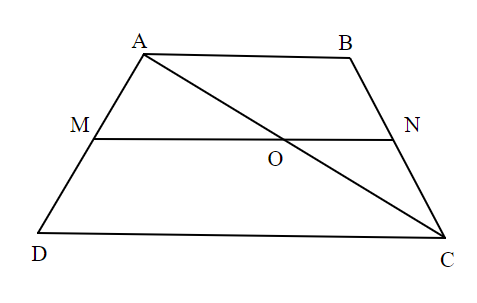Courses
Courses for Kids
Free study material
Free LIVE classes
MoreLIVE
Join Vedantu’s FREE Mastercalss

# In trapezium ABCD, $M \in \overline {AD} ,N \in \overline {BC}$ are the points such $\dfrac{{AM}}{{MD}} = \dfrac{{BN}}{{NC}} = \dfrac{2}{3}$. The diagonal $\overline {AC}$ intersects $\overline {MN}$ at$O$. Then find the value of $\dfrac{{AO}}{{AC}}$.Verified
362.1k+ views
Hint: A trapezium is a 2D shape which falls under the category of quadrilaterals. A trapezium has two parallel sides and two non-parallel sides. Using this information first draw the diagram with the given data and solve the problem accordingly.

In the given trapezium ABCD, $\overline {AB} \parallel \overline {CD}$, $M$ and $N$are the points on the traversals $\overleftrightarrow {AD}$ and $\overleftrightarrow {BC}$ respectively as shown in the below diagram.Also, given $\dfrac{{AM}}{{MD}} = \dfrac{{BN}}{{NC}} = \dfrac{2}{3}$
So, clearly from the diagram, $\overline {MN} \parallel \overline {AB}$ and $\overline {AB} \parallel \overline {CD}$.
Given that diagonal $\overline {AC}$ intersects $\overline {MN}$ at $O$.
In $\Delta ADC$, $\overline {MO} \parallel \overline {DC}$ such that $M \in \overline {AD} {\text{ }}\& {\text{ }}O \in \overline {AC}$.
We know that by the Triangle Proportionality theorem, if a line parallel to one side of a triangle intersects the other two sides of the triangle, then the lines divide these two sides proportionally.
By using this property in $\Delta ADC$, we have
$\Rightarrow \dfrac{{AM}}{{MD}} = \dfrac{{AO}}{{OC}}$
But we have $\dfrac{{AM}}{{MD}} = \dfrac{2}{3}$
So, $\dfrac{{AO}}{{OC}} = \dfrac{2}{3}$
We have to find $\dfrac{{AO}}{{AC}}$. From the diagram, $OC = AO + OC$
$\Rightarrow \dfrac{{AO}}{{OC}} = \dfrac{{AO}}{{AO + OC}} \\ \Rightarrow \dfrac{{AO}}{{OC}} = \dfrac{2}{{2 + 3}} \\ \therefore \dfrac{{AO}}{{OC}} = \dfrac{2}{5} \\$
Thus, $\dfrac{{AO}}{{OC}} = \dfrac{2}{5}$.

Note: The length of the mid-segment is equal to half of the sum of parallel bases in a trapezium. In this problem we have used both the properties of triangles as well as the properties of trapezium.
Last updated date: 01st Oct 2023
Total views: 362.1k
Views today: 5.62k